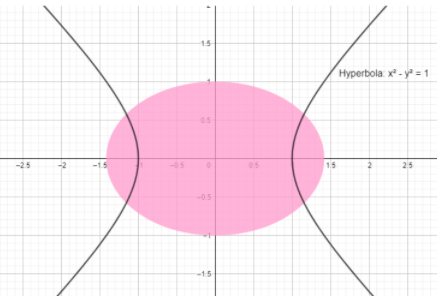# An ellipse intersects the hyperbola $2{{x}^{2}}-2{{y}^{2}}=1$ orthogonally. The eccentricity of the ellipse is reciprocal of that of the hyperbola. If the axes of the ellipse are along the coordinate axes, then(This question has multiple correct options)(a) equation of ellipse is ${{x}^{2}}+2{{y}^{2}}=2$ (b) the foci of the ellipse are (±1, 0) (c) equation of ellipse is ${{x}^{2}}+2{{y}^{2}}=4$(d) the foci of ellipse are $\left( \pm \sqrt{2},0 \right)$Verified
203.1k+ views
Hint: To understand the question better, we shall draw the figure of the hyperbola given to use. We shall also draw a general ellipse. It is given that the axes of the ellipse are coordinate axes. This means the center of the ellipse is the origin (0, 0). It is given that ellipse and hyperbola intersect orthogonally. This means, at their point of intersection, tangents to the conics are perpendicular to each other. From the given conditions, we shall try to find the equation of the ellipse.

Complete step-by-step solution:
The hyperbola given to us is $2{{x}^{2}}-2{{y}^{2}}=1$. We also know that the ellipse has coordinate axes as its major and minor axes.
Thus, we will draw a figure of the above-mentioned conics.As we can see from the equation and figure, we know that the hyperbola is a rectangular hyperbola.
Thus, the eccentricity of the rectangular hyperbola is always $\sqrt{2}$.
It is given that the eccentricity of the ellipse is reciprocal as that of the hyperbola.
Thus, eccentricity e of ellipse is $\dfrac{1}{\sqrt{2}}$.
Now, as we know that the centre of the ellipse coincides with the origin (0, 0). The general equation of an ellipse centred at origin is $\dfrac{{{x}^{2}}}{{{a}^{2}}}+\dfrac{{{y}^{2}}}{{{b}^{2}}}=1$, where ${{b}^{2}}={{a}^{2}}\left( 1-{{e}^{2}} \right)$.
But e = $\dfrac{1}{\sqrt{2}}$
\begin{align} & \Rightarrow {{b}^{2}}={{a}^{2}}\left( 1-\dfrac{1}{2} \right) \\ & \Rightarrow {{b}^{2}}=\dfrac{{{a}^{2}}}{2} \\ \end{align}
Thus, the equation of the ellipse is $\dfrac{{{x}^{2}}}{{{a}^{2}}}+\dfrac{{{y}^{2}}}{\dfrac{{{a}^{2}}}{2}}=1\Rightarrow {{x}^{2}}+2{{y}^{2}}={{a}^{2}}$.
Let $\left( {{x}_{1}},{{y}_{1}} \right)$ be the point of intersection of the ellipse ${{x}^{2}}+2{{y}^{2}}={{a}^{2}}$ and hyperbola $2{{x}^{2}}-2{{y}^{2}}=1$.
The equation of the tangents at point $\left( {{x}_{1}},{{y}_{1}} \right)$ on different conics is as follows:
Ellipse: ${{x}_{1}}x+2{{y}_{1}}y={{a}^{2}}$ and slope: $-\dfrac{{{x}_{1}}}{2{{y}_{1}}}$
Hyperbola: $2{{x}_{1}}x-2{{y}_{1}}y=1$ and slope: $\dfrac{{{x}_{1}}}{{{y}_{1}}}$
As the hyperbola and ellipse are orthogonal to each other, the tangents are perpendicular to each other. Thus, product of their slopes is -1.
\begin{align} & \Rightarrow \left( -\dfrac{{{x}_{1}}}{2{{y}_{1}}} \right)\left( \dfrac{{{x}_{1}}}{{{y}_{1}}} \right)=-1 \\ & \Rightarrow x_{1}^{2}=2y_{1}^{2} \\ \end{align}
If $\left( {{x}_{1}},{{y}_{1}} \right)$ is the point of intersection, it lies on the ellipse and on the hyperbola.
Thus $x_{1}^{2}+2y_{1}^{2}={{a}^{2}}$ and $2x_{1}^{2}-2y_{1}^{2}=1$
Substitute $x_{1}^{2}=2y_{1}^{2}$ in $2x_{1}^{2}-2y_{1}^{2}=1$
\begin{align} & \Rightarrow 2\left( 2y_{1}^{2} \right)-2y_{1}^{2}=1 \\ & \Rightarrow 2y_{1}^{2}=1 \\ & \Rightarrow y_{1}^{2}=\dfrac{1}{2} \\ & \Rightarrow x_{1}^{2}=2y_{1}^{2} \\ & \Rightarrow x_{1}^{2}=2\left( \dfrac{1}{2} \right) \\ & \Rightarrow x_{1}^{2}=1 \\ \end{align}
Substitute $y_{1}^{2}=\dfrac{1}{2}$ and $x_{1}^{2}=1$ in the equation $x_{1}^{2}+2y_{1}^{2}={{a}^{2}}$
\begin{align} & \Rightarrow 1+2\left( \dfrac{1}{2} \right)={{a}^{2}} \\ & \Rightarrow {{a}^{2}}=2 \\ \end{align}
Therefore, the equation of the ellipse is ${{x}^{2}}+2{{y}^{2}}=2$.
Thus, option (a) is the correct option.
The foci of ellipse with origin as centre is $\left( \pm ae,0 \right)$.
a = $\sqrt{2}$ and e = $\dfrac{1}{\sqrt{2}}$
Thus, foci of the ellipse is $\left( \pm \sqrt{2}\times \dfrac{1}{\sqrt{2}},0 \right)=\left( \pm 1,0 \right)$
Hence, option (b) is also the correct option.

Note: The eccentricity of a hyperbola is always greater than 1 and can be found by the relation $e=\dfrac{\sqrt{{{a}^{2}}+{{b}^{2}}}}{a}$. For a rectangular hyperbola, a = b. Thus, the eccentricity of a rectangular hyperbola is $\sqrt{2}$. The equation of tangents can be found by derivation of the equation of the conic, then replacing dx by x and dy by y. The x and y are replaced by x and y coordinates of the point at which tangent has to be found. It is to be noted that the derivation of constant is not carried out. For example, the equation of a rectangular hyperbola is $\dfrac{{{x}^{2}}}{{{a}^{2}}}-\dfrac{{{y}^{2}}}{{{b}^{2}}}=1$, so equation of tangent at point (m, n) will be $\dfrac{2mx}{{{a}^{2}}}-\dfrac{2ny}{{{b}^{2}}}=1$.